# MiniApps

The following are a collection of miniApps we have implemented to demonstrate the efficacy of the Charm++ model:

## LeanMD

LeanMD is a molecular dynamics simulation program written in Charm++. This benchmark simulates the behavior of atoms based on the Lennard-Jones potential, which is an effective potential that describes the interaction between two uncharged molecules or atoms. The computation performed in this code mimics the short-range non-bonded force calculation in NAMD, and resembles the LJ force computation in miniMD benchmark in the Mantevo benchmark suite maintained by Sandia National Laboratories.

The force calculation in Lennard-Jones dynamics is done within a cutoff-radius, rc for every atom. In LeanMD, the computation is parallelized using a hybrid scheme of spatial and force decomposition. The three-dimensional (3D) simulation space consisting of atoms is divided into cells of dimensions that are equal to the sum of the cutoff distance, rc and a margin. In each iteration, force calculations are done for all pairs of atoms that are within the cutoff distance. The force calculation for a pair of cells is assigned to a different set of objects called computes. Based on the forces sent by the computes, the cells perform the force integration and update various properties of their atoms – acceleration, velocity and positions.

Features: Automated load balancing, fault tolerance, multicast manager.

## AMR

AMR is mesh restructuring algorithm for adaptive mesh refinement computations. The parallel mesh restructuring algorithm operates in terms of near-neighbor communication among individual blocks, and a single synchronization-only collective.

Traditional AMR algorithms phrase the design in terms of processors that contain many blocks. Instead, we promote blocks to first-class entities that act as a virtual processor. As the mesh is refined or coarsened in AMR, the number of blocks will change and traditional algorithms require synchronization at these points. However, to enhance productively, we abstract the blocks as a collection that dynamically expands and contracts over time. Refinement decisions can then be local to each block and propagated as far as algorithmically required so blocks are always within one refinement level of their neighbors. Because remeshing occurs only at discrete points in the simulation time, instead of using collective communication that is proportional to the depth of the recursive refinement, we use a scalable termination detection mechanism built into our runtime to globally determine when all refinement decisions have been finalized. Previous collective methods require O(d) rounds of collective communication, where d is the refinement and consume O(P) memory per processor to store the results. By utilizing termination detection, we consume a negligible amount of memory and communicate no data. Besides termination detection, blocks execute completely asynchronously, communicating only with neighboring blocks when required.

Traditional AMR implementations store the quad-tree instance on each process consuming O(P) memory and taking O(log N) time for neighbor lookup. We organize the blocks into a quad-tree but address each block by their location in the tree using bit vectors to represent quadrants recursively. It requires only O(#blocks/P) memory per process and O(1) lookup time. It also frees the programmer from having to know where the block lies; instead, the underlying runtime system manages the physical locations of each block and provides direct, efficient communication between them. The runtime system can then redistribute the blocks periodically without any change to the logic.

Features: quiescence detection, dynamic chare creation, load balancing.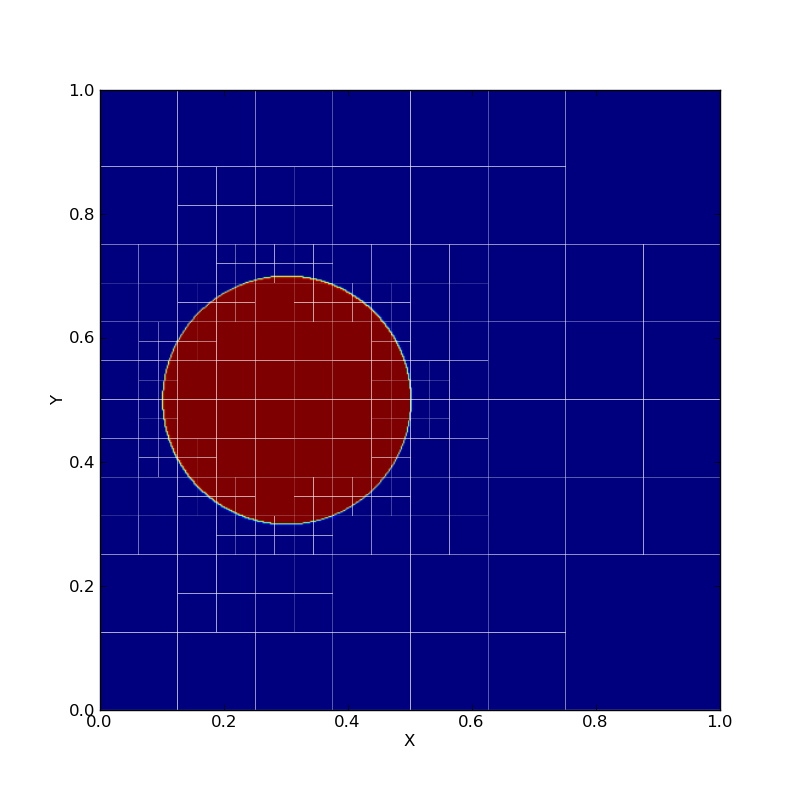Figure: example simulation of a circular fluid advected by a constant velocity field. From left to right, the figure displays the fluid density after 2, 572, and 1022 iterations respectively. The white squares demonstrate how the mesh is evolving over time.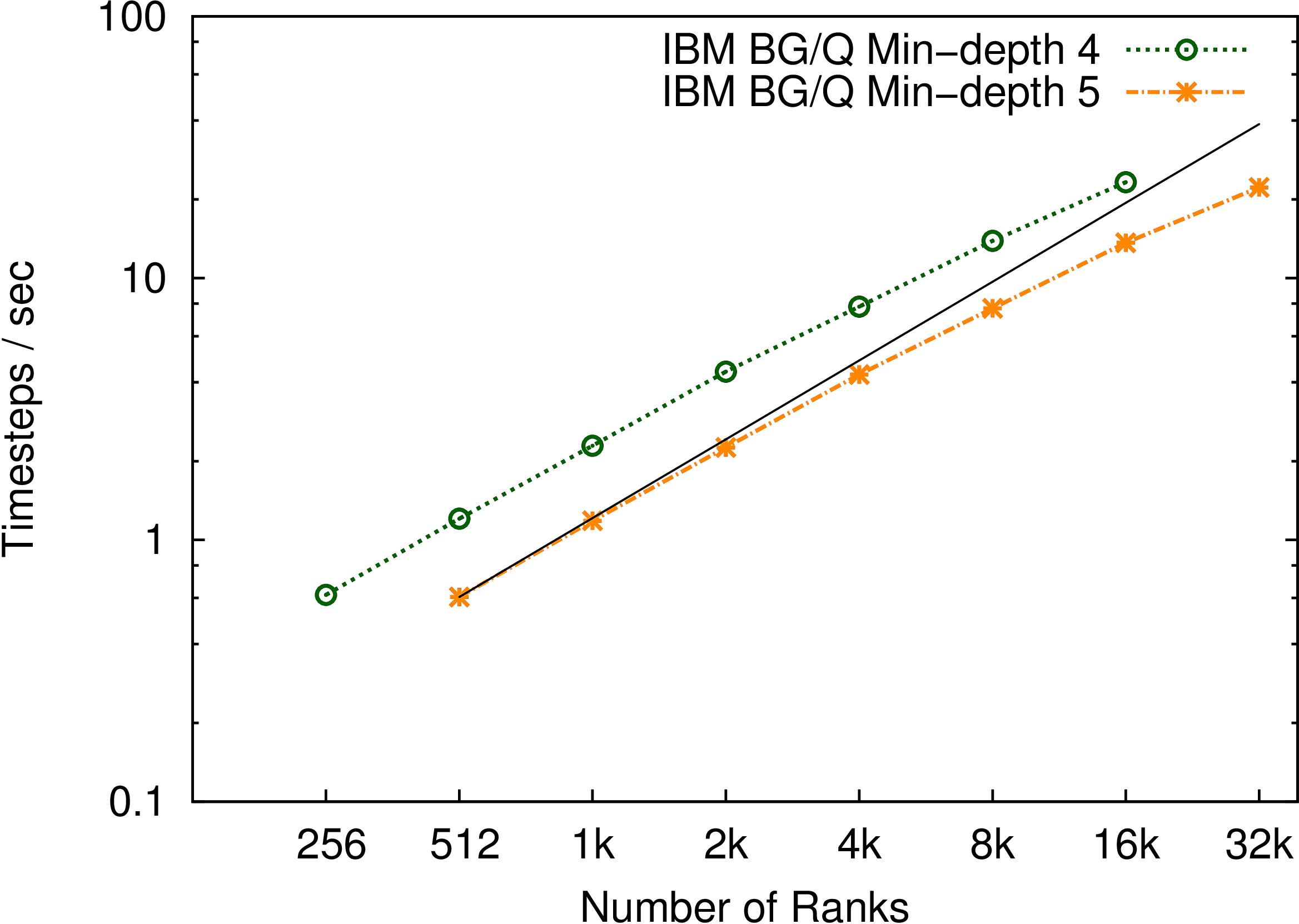Figure: performance of AMR on Blue Gene/Q. Left: Timesteps per second strong scaling on IBM BG/Q with a max depth of 15. Right: The non-overlapped delay of remeshing in milliseconds. The candlestick graphs the minimum and maximum values, the 5th and 95th percentile, and the median.

## Barnes-Hut

The Barnes-Hut algorithm is a tree-based scheme to compute fast and approximate time-series solutions to the N-body problem, where Barnes-Hut algorithm for N-body problem is a method of calculating forces on a system of N bodies that grows only as N log N. It uses a tree-structured hierarchical recursive subdivision of space into cubic cells. Barnes-Hut method is widely used in cosmological simulations.

The N-body problem involves the numerical calculation of the trajectories of N point masses (or charges) moving under the influence of a conservative force field such as that induced by gravity (or electrical charges). In its simplest form, the method models bodies as particles of zero extent moving in a collision-less manner. The objective is to calculate the net force incident on every particle at discrete time steps. These forces are then used to update the velocity and position of each particle, leading into the next time step, where the net force on each particle is calculated once more, etc. In general, the force may be long-range in nature (as is the case with gravity), so that interactions between distant particles must also be calculated. Thus, in order to obtain a good approximation to the actual solution of a system, O(N2) computations must be performed. Given its quadratic complexity, the amount of work done by this all-pairs method makes it infeasible for systems with large N.

Barnes and Hut devised a hierarchical N-body method that performs significantly fewer computations but at the cost of a greater relative error in the computed solution. The method relies on the spatial partitioning of the input system of particles, thereby imposing a tree-structure on it. Particles that are close to each other in space are grouped into closely related nodes of the tree. This allows the approximation of forces on a particle due to a distant group of particles through the multipole moments of that group. Note that applying such an approximation to points relatively close to the group will result in gross errors of calculation. In such a case, sub-partitions within the group are tested for proximity to the point. This technique, applied systematically, yields an expected complexity of O(N lg N), making it suitable for large systems of particles.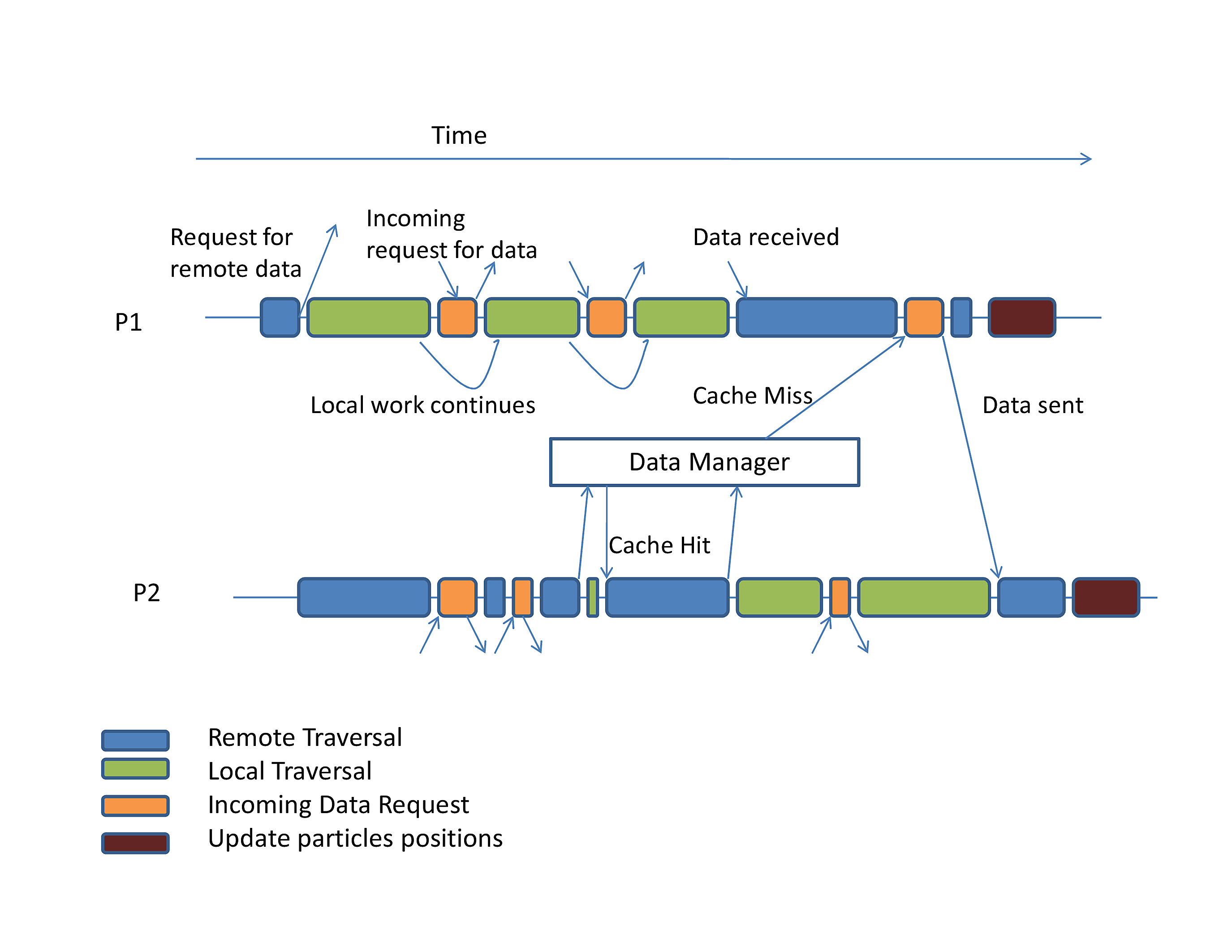Figure: Time Progression view showing 2 processors executing an iteration of Barnes-Hut.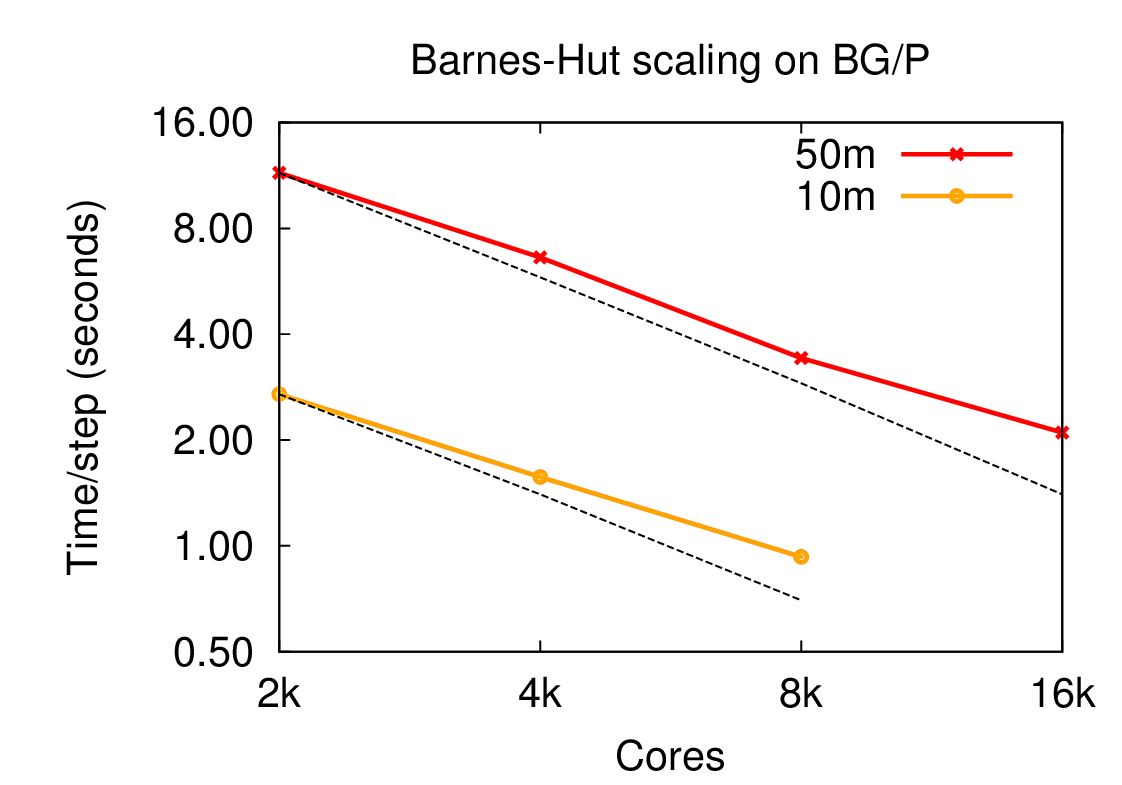Figure: Performance of Barnes-Hut for 10 and 50 million particle systems on Intrepid (IBM BG/P).

## Dense LU

This benchmark is the Charm++ implementation of LU solver, one of the seven HPC Challenge miniApps. Since Charm++ is a general and fully capable programming paradigm, our LU implementation does not employ any linear algebra specific notations. Our implementation is very succinct and presents several distinct benefits.

We use a typical block-decomposed algorithm for the LU factorization process. Our focus in this effort has been less on choosing the best possible factorization algorithm than on demonstrating productivity with a reasonable choice. The input matrix of n x n elements is decomposed into square blocks of b x b elements each. We delegate underlying sequential operations to an available high performance linear algebra library, typically a platform-specific implementation of BLAS and perform partial pivoting for each matrix column.

Features: composable library, flexible data placement, block-centric control flow, separation of concerns.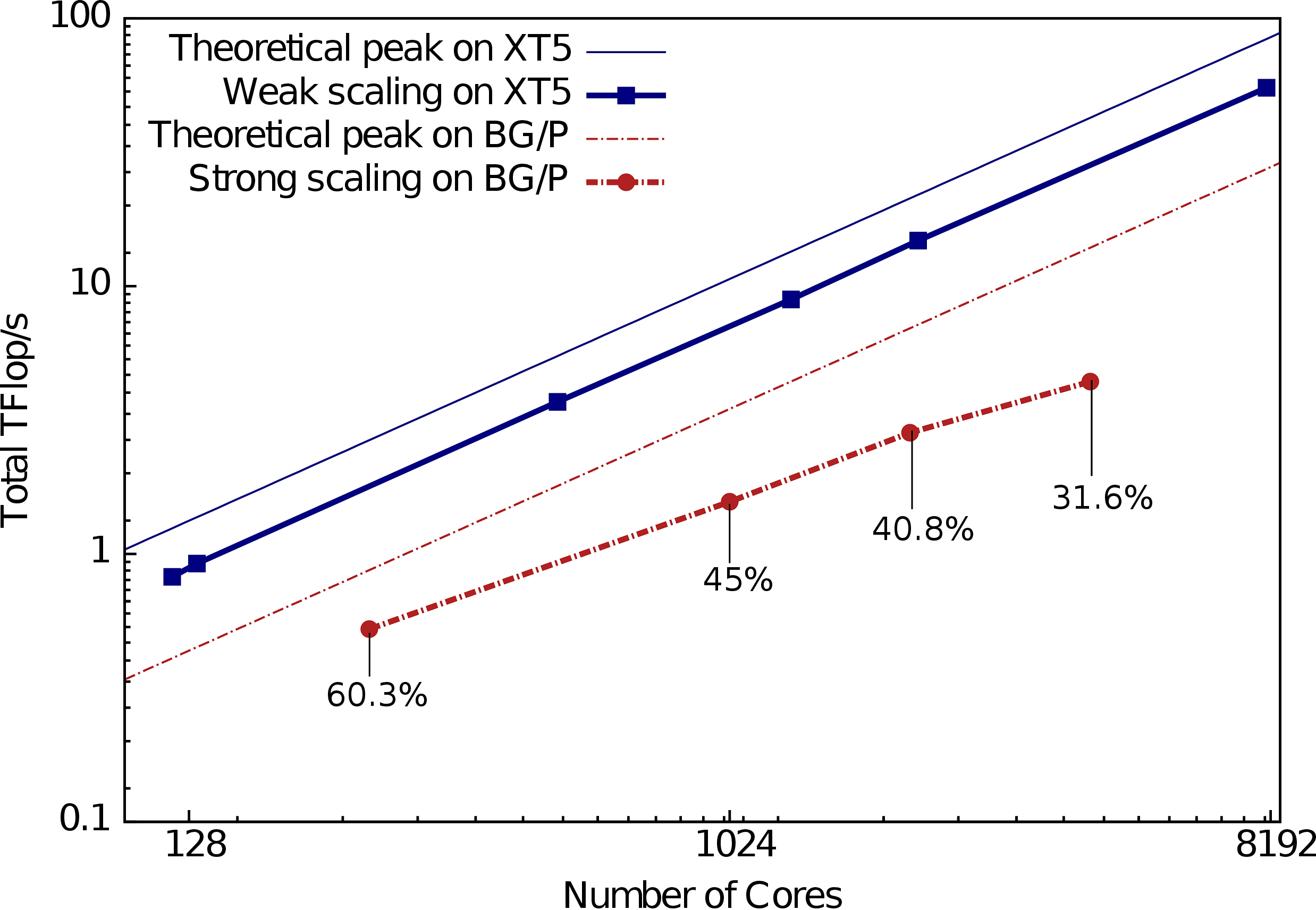Figure: weak scaling (matrix occupies 75% of memory) from 120 to 8064 processors on Jaguar (Cray XT5). Strong scaling (n = 96, 000) from 256 to 4096 processors on Intrepid (IBM BG/P).

## HPCCG

HPCCG is Charm++ implementation of HPCCG mini-application in the Mantevo Suite. It was originally developed as the first Mantevo mini-app in order to be the best representation of an unstructured implicit finite element or finite volume application in 800 lines of code.

## Kripke

Kripke is a proxy application from Lawrence Livermore National Laboratory (LLNL) for Sn discrete particle transport codes. Given a volume of interest, knowledge of its boundary conditions and the particle-generating sources within and outside the domain, Kripke solves for the flux of particles at every point in the domain at a subsequent time. It does so by decomposing the domain into 3-dimensional subdomains that we call zone sets, and then sweeps the over the zone sets for all energy group sets and angular direction sets. These parallel sweeps are all independent of each other, and so Kripke sweeps from all corners of the domain simultaneously and pipelines sweeps originating from the same domain corner.

## Triangular Solver

Solution of sparse triangular systems of linear equations is a performance bottleneck in many methods for solving more general sparse systems, such as many iterative methods with preconditioners. In our algorithm, the matrix is divided into blocks of columns, where each block is analyzed to find its independent rows for solution. In addition, if there are dense regions below the diagonal section (we assume lower triangular for description), they are divided into new blocks. Each diagonal block starts the computation with its independent parts and waits for its dependency messages from the left. Nondiagonal blocks wait for the solution values from their corresponding diagonal block, and then start their computation.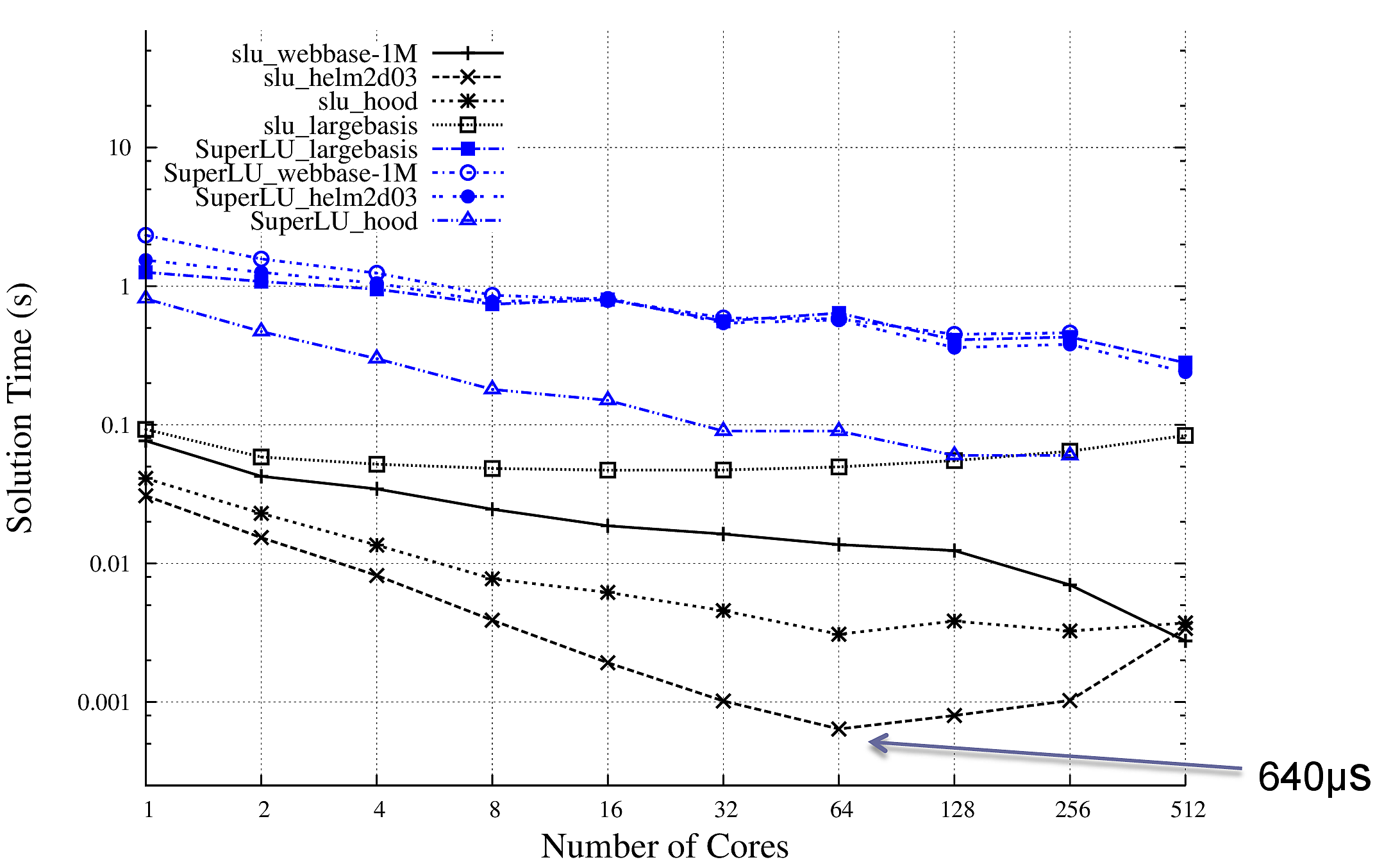Figure: performance of sparse triangular solver on Intrepid (IBM BG/P) for different sparse matrices. Performance is much better than the standard SuperLU DIST package.

## 1D FFT

Our implementation of Global FFT takes input size N and performs a complex 1D FFT on an NxN matrix where subsequent rows are contiguous data elements of a double precision complex vector. Three all-to-all transposes are required to perform the FFT and unscramble the data. All-to-all operations are executed via point-to-point messages and external libraries (FFTW or ESSL) perform serial FFTs on the rows of the matrix.

Features: interoperability with MPI, adaptive overlap.

## Random Access

The HPC Challenge Random Access benchmark measures the rate of processing updates to a distributed table. Each process issues updates to random locations in the table. The small size of individual update messages in this benchmark makes it prohibitively expensive to send each item as a separate message. This makes the benchmark an ideal scenario for using TRAM, our general purpose message aggregation library. TRAM routes messages over an N-dimensional virtual topology comprising the PEs involved in the run. The topology needs to be specified when creating an instance of the library. To facilitate the specification of the virtual topology, we used the Charm++ Topology Manager library, which provides an interface to determine the network topology for the current run. In typical cases, we found that using virtual topologies with dimensions matching the network representation of the current run led to good performance.

We used a Charm++ group to partition the global table across the nodes in a run. Each element of the group allocates its part of the global table, generates random update keys, and sends the updates to the appropriate destination.

In the context of TRAM, each processor is limited to sending to and receiving messages from a subset of the processors involved in the run. When determining where to send a particular data item (in our case table update), TRAM selects a destination from among its peers so that data items always make forward progress toward the destination. Items traveling to the same intermediate destination are combined into larger messages. This approach achieves improved aggregation and lower memory utilization compared to schemes which aggregate separately for each destination on the network.

Features: TRAM, automated topology discovery.Figure: Random access in Charm++. Left: message aggregation using TRAM. Right: performance of random access on Intrepid (IBM BG/P) and Vesta (IBM BG/Q).

## EP Stream

This benchmark is a simple Charm++ implementation of the HPC Challenge stream benchmark.